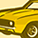cancel
Showing results for
Did you mean:
cancel
Showing results for
Did you mean:
Developer forum

## Setting array dimensions with parametersEnthusiast
Hi

I'm trying to set an array's dimension by using a parameter value, like this:
```num = 3 !will be set by the parameter

DIM myArray[num] ```
This gives an error as: "Array dimensions must be positive numeric constants ..."

It does work, of course, with: DIM myArray , but I want this array-dimension to be based on a parameter.

How to achieve this?

Thanks.
8 REPLIES 8Ace
Archive it different way.
```
num=3

DIM myArray[]

FOR i=1 to num

myArray=something

NEXT i
```Expert
best practice is to build a local array and pass it to to the parameter array, and then also use/reference this local array (assuming master script) in other scripts instead of the parameter array as this means you don't have to wait for the parameter to update in the array for it to apply in the code because the local array already has the value from the master script which is run first.

```
num = 3

dim _myArray[]
for i = 1 to num
if i > vardim1(myArray) then
_myArray = "put a default value here"
else
_myArray = myArray
endif
next i
myArray = _myArray
parameters myArray = myArray
```
Creator of Cadswift's parametric GDL libraries
Creator of Infinite Openings and Component Catalogues
Push the envelope & watch it bendAce
Kristian wrote:
best practice is to build a local array and pass it to to the parameter array, and then also use/reference this local array (assuming master script) in other scripts instead of the parameter array as this means you don't have to wait for the parameter to update in the array for it to apply in the code because the local array already has the value from the master script which is run first.

```
num = 3

dim _myArray[]
for i = 1 to num
if i > vardim1(myArray) then
_myArray = "put a default value here"
else
_myArray = myArray
endif
next i
myArray = _myArray
parameters myArray = myArray
```
It depends on what you need your array. I'm using the method you describe when I have an array (let say coordinates of polygon) and I want to add a new node. Then if I have an array with three points coordinates, I'm passing their coordinates to temporary array, adding at the end additional dimension and coordinates of the new point.

I also can say - I've found many GDL scripts (especially from ArchiCAD library) are very very big. They writing with 20-30 lines that potentially possible to write with 10. I don't know where is the secret in it - is it example of good programming Graphisoft is showing to us (well they are mathematicians, studied programming in university) - but somehow I have decided to develop different style trying to make scripts as short as possible. And also that script possible to read by human - without additional notes and explanations, so I'm paying extra attention to variable names.Expert
Podolsky wrote:
It depends on what you need your array. I'm using the method you describe when I have an array (let say coordinates of polygon) and I want to add a new node
I agree completely. You should be as efficient as possible in your code. Most of my arrays require the dynamic variation so that is the method I apply. If your application does not need to be dynamic like editing a polygon then perhaps it is overkill.
Creator of Cadswift's parametric GDL libraries
Creator of Infinite Openings and Component Catalogues
Push the envelope & watch it bendEnthusiast
Thanks, Podolsky & Kristian.

Podolsky, yes, that looks like a way around it.

How do you initialise a two-dimensional array as a blank? DIM myArray[][] ?Ace
dushyant wrote:
Thanks, Podolsky & Kristian.

Podolsky, yes, that looks like a way around it.

How do you initialise a two-dimensional array as a blank? DIM myArray[][] ?
yesEnthusiast
Thanks!Once I had similar issue - that's the reply of Peter Baksa
AC 22, 24 | Win 10Latest solutions

Most liked posts